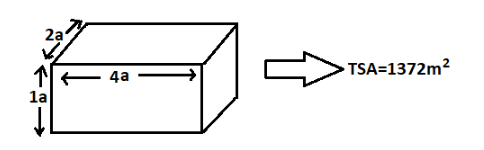The length, breadth and height of a cuboid are in the ratio 4:2:1 and its total surface area is. Find the dimensions of the cuboid.

# The length, breadth and height of a cuboid are in the ratio 4:2:1 and its total surface area is. Find the dimensions of the cuboid.

1. A2. B3. C4. DFill Out the Form for Expert Academic Guidance!l

+91

Live ClassesBooksTest SeriesSelf Learning

Verify OTP Code (required)

### Solution:

We are given that the total surface area of a cuboid isand the ratio of its length to breadth to height is 4:2:1. We have to find the dimensions of the cuboid.
Let the length be 4a m, breadth be 2a m and height be 1a m.Total surface area of the cuboid is.We are already given that TSA of the cuboid is.
This meansWe have got the value of  7 m.
Therefore length is 4a m which is equal to, breadth is 2a m which isand height is 1a m which is.
Therefore, the dimensions of the cuboid are.
Hence, option 2 is the correct Ans:.

## Related content

 Area of Square Area of Isosceles Triangle Pythagoras Theorem Triangle Formula Perimeter of Triangle Formula Area Formulae Volume of Cone Formula Matrices and Determinants_mathematics Critical Points Solved Examples Type of relations_mathematics+91

Live ClassesBooksTest SeriesSelf Learning

Verify OTP Code (required)